# 强制约束中数值问题的处理方法

2018年 9月 11日

### 对全局约束使用拉格朗日乘子法

E[u(x)] = \int_a^b u(x)\sqrt{1+u^{\prime}(x)^2}dx.

\int_a^b \sqrt{1+u^{\prime}(x)^2}dx = l.

\mathcal{L}[u(x),\lambda] = \int_a^b u(x)\sqrt{1+u^{\prime}(x)2}dx + \lambda \left[\int_a^b \sqrt{1+u^{\prime}(x)^2}dx – l \right].

F = u(x)\sqrt{1+u^{\prime}(x)2}, \qquad g = \sqrt{1+u^{\prime}(x)^2}

[u(x),\lambda]

\int_a^b \left[\frac{\partial F}{\partial u}\hat{u} + \frac{\partial F} {\partial u’}\hat{u’}\right]dx + \lambda\int_a^b \left[\frac{\partial g}{\partial u}\hat{u} + \frac{\partial g}{\partial u’}\hat{u’}\right]dx=0, \quad \forall \hat{u},
\hat{\lambda}\left[\int_a^b g(x,u,u’)dx – l \right]=0, \quad \forall \hat{\lambda}.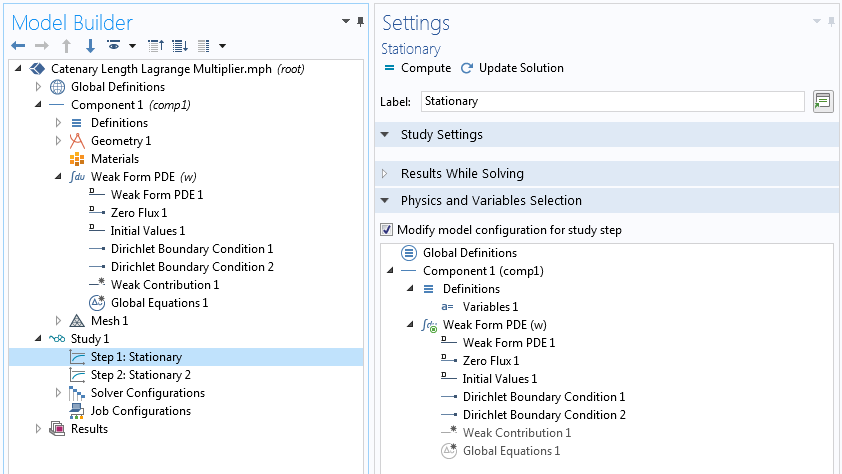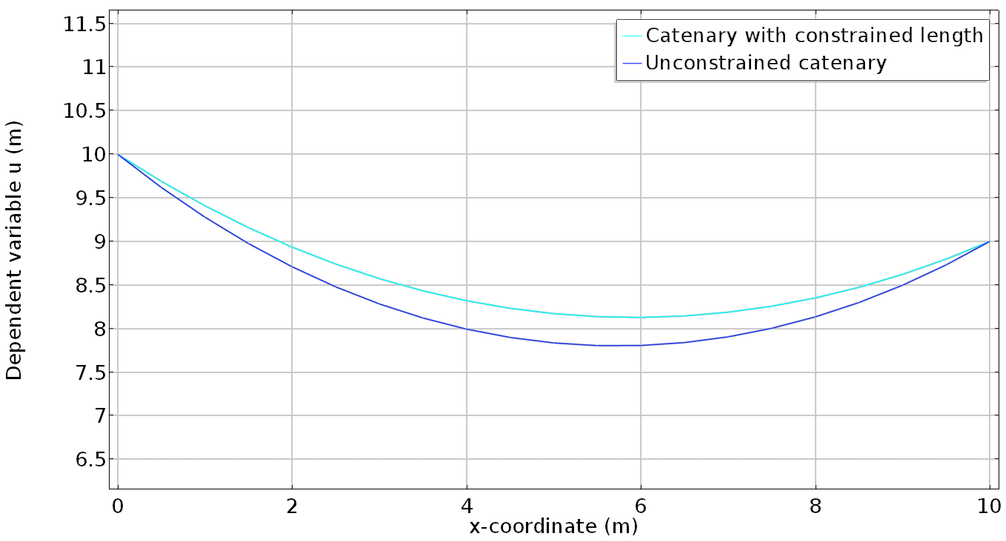### 罚方法

E_{\mu}[u(x)] = \int_a^b F(x,u,u^{\prime})dx + \frac{\mu}{2} \left[\int_a^b g(x,u,u’)dx – l \right]^2.

\int_a^b \left[\frac{\partial F}{\partial u}\hat{u}+\frac{\partial F}{\partial u’}\hat{u’}
\right]dx + \mu \left[\int_a^b g(x,u,u’)dx – l \right]\int_a^b \left[\frac{\partial g}{\partial u}\hat{u}+\frac{\partial g} {\partial u’}\hat{u’}\right]dx, \qquad \forall \hat{u}.

\lambda \approx \mu \left[\int_a^b g(x,u,u’)dx – l \right].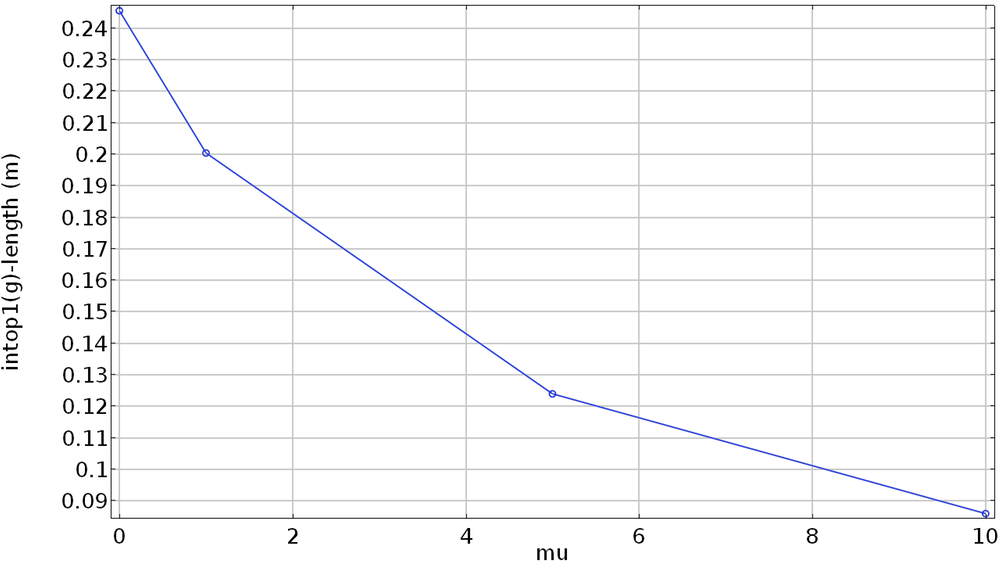#### 罚参数的辅助扫描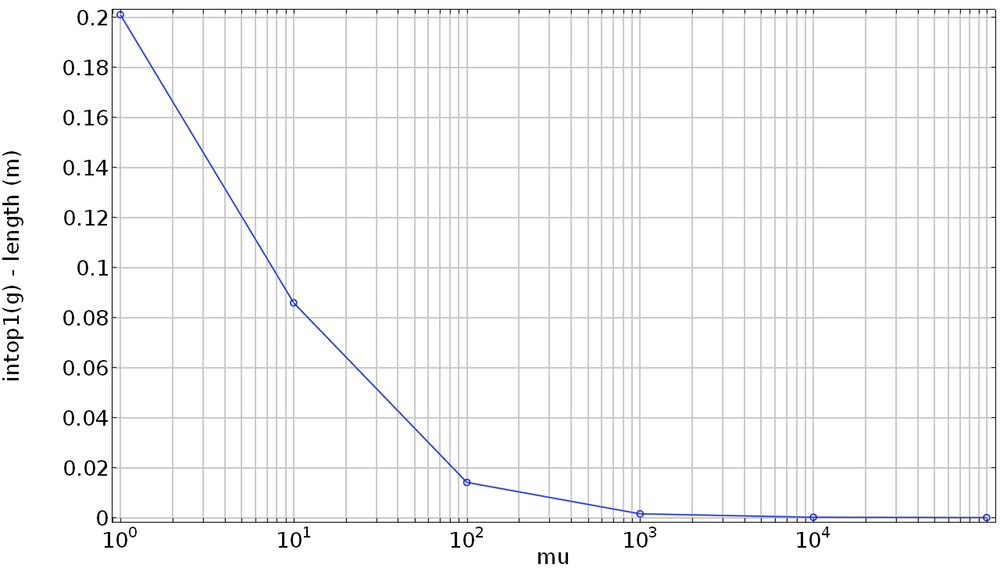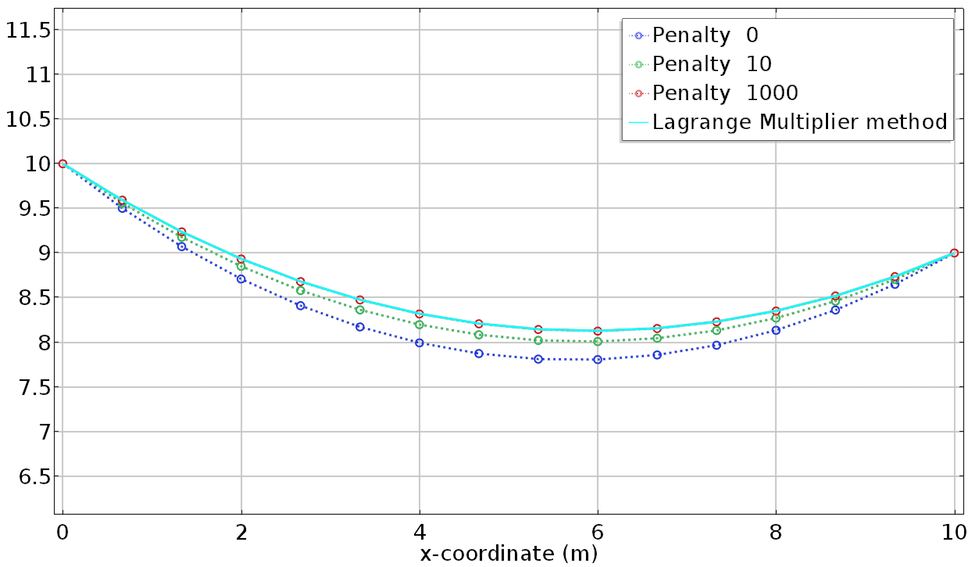### 增广拉格朗日法

\textrm{minimize } f(x), \quad \textrm{subject to } g(x)=0.

\mathcal{L}(x,\lambda) = f(x) + \lambda g(x),

E_{\mu}(x) = f(x) + \frac{\mu}{2}g^2(x).

E_{\mu,\lambda}(x) = f(x) + \lambda^* g(x) + \frac{\mu}{2}g^2(x).

\frac{d}{dx} E_{\mu,\lambda} = \frac{df}{dx} + \lambda^* \frac{dg}{dx} +\mu g \frac{dg}{dx} = \frac{df}{dx} + (\lambda^* +\mu g )\frac{dg}{dx} = 0.

\lambda = \lambda^* +\mu g .

\int_a^b \left[\frac{\partial F}{\partial u}\hat{u}+\frac{\partial F}{\partial u’}
\hat

{u’}\right]dx +
\left(\lambda_k + \mu \left[\int_a^b g(x,u,u’)dx – l \right]\right)
\int_a^b \left[\frac{\partial g}{\partial u}\hat{u}+\frac{\partial g}{\partial u’}\hat{u’}

{u}
.

\lambda_
{k+1}
=\lambda_k + \mu \left[\int_a^b g(x,u,u’)dx – l \right]